Chapter 27 - Organization of memory

The memory is sorted into different areas for storing different kinds of information. The areas are only large enough for the information that they actually contain, & if you insert some more at a given point (for instance by adding a program line or variable) space is made by shifting up everything above that point. Conversely, if you delete information then everything above it is shifted down.The system variables contain various pieces of information that tell the computer what sort of state the computer is in. They are listed fully in the next chapter, but for the moment note that there are some (called D_FILE, VARS, E_LINE & so on) that contain the addresses of the boundaries between the various areas in the memory. These are not BASIC variables, & their names will not be recognized by the computer.

Each line in the program is stored as: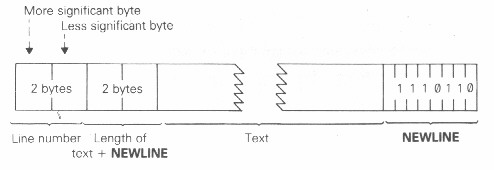Note that, in contrast with all other cases of two-byte numbers in the Z80, the line number here (& also in a FOR-NEXT control variable) is stored with its most significant byte first: that is to say, in the order that you would write them down in.

A numerical constant in the program is followed by its binary form, using the character CHR\$ 126 followed by five bytes for the number itself.

The display file is the memory copy of the television picture. It begins with a NEWLINE character, & then has the twenty four lines of text, each finishing with a NEWLINE. The system is so designed that a line of text does not need space a full thirty two characters: final spaces can be omitted. This is used to save space when the memory is small.

When the total amount of memory (according to the system variable RAMTOP) is less than 3 1/4 K, then a clear screen - as set up at the start or by CLS - consists of just twenty five NEWLINEs. When the memory is bigger than a clear screen is padded out with 24*32 spaces & on the whole it stays at its full size; SCROLL, however, & certain conditions where the lower part of the screen expands to more than two lines, can upset this by introducing short lines at the bottom.

The variables have different formats according to their different natures.

Number whose name is one letter only: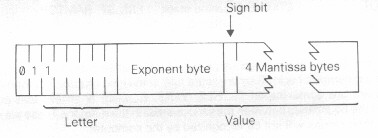Number whose name is longer than one letter: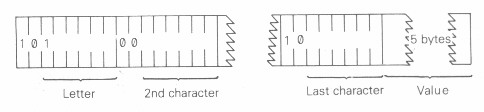Array of numbers:The order of the elements is:

first, the elements for which the first subscript is 1

next, the elements for which the first subscript is 2

next, the elements for which the first subscript is 3

& so on for all possible values of the first subscript.

The elements with a given first subscript are ordered in the same way using the second subscript, & so on down to the last.

As an example, the elements of the 3 x 6 array B in chapter 22 are stored in the order

B(1,1),B(1,2),B(1,3),B(1,4),B(1,5),B(1,6),B(2,1),B(2,2),...,B(2,6),B(3,1),B(3,2),...,B(3,6).

Control variable of a FOR-NEXT loop: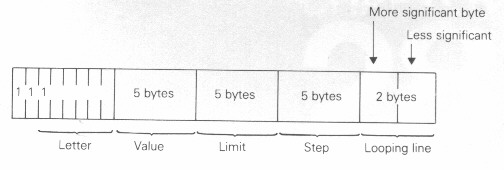String: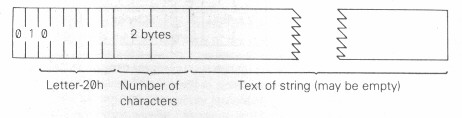Array of characters:The part string at E_LINE contains the line being typed (as a command, a program line, or INPUT data) & also some work space.

The calculator is the part of the BASIC system that deals with arithmetic, & the numbers on which it is operating are held mostly in the calculator stack.

The spare part contains the space so far unused.

The machine stack is the stack used by the Z80 chip to hold return addresses & so on.

The GOSUB stack was mentioned in chapter 14.

The space for USR routines has to be set aside by you, using NEW as described in the last chapter.

Previous: Chapter 26    Next: Chapter 28